# Deep Learning — Different Types of Autoencoders

In this post we will understand different types of Autoencoders

Read here to understand what is Autoencoder, how does Autoencoder work and where are they used.

Just quick brief on what is Autoencoders

Autoencoders encodes the input values x using a function f. Then decodes the encoded values f(x) using a function g to create output values identical to the input values.

Autoencoder objective is to minimize reconstruction error between the input and output. This helps autoencoders to learn important features present in the data. When a representation allows a good reconstruction of its input then it has retained much of the information present in the input.

### What are different types of Autoencoders?

#### Undercomplete Autoencoders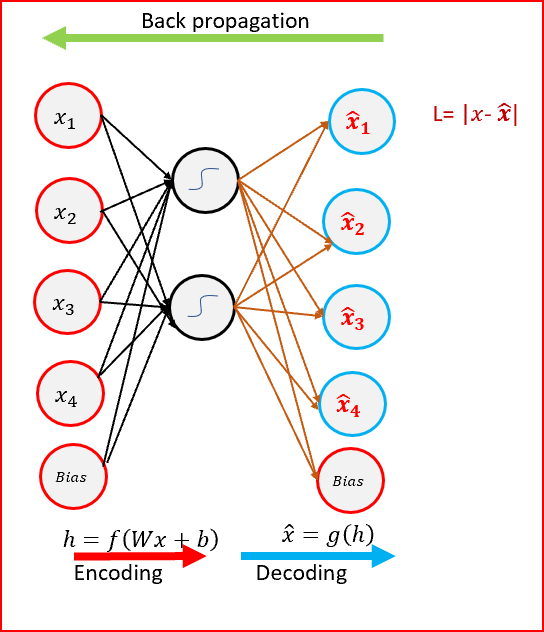Undercomplete Autoencoder- Hidden layer has smaller dimension than input layer
• Goal of the Autoencoder is to capture the most important features present in the data.
• Undercomplete autoencoders have a smaller dimension for hidden layer compared to the input layer. This helps to obtain important features from the data.
• Objective is to minimize the loss function by penalizing the g(f(x)) for being different from the input x.
• When decoder is linear and we use a mean squared error loss function then undercomplete autoencoder generates a reduced feature space similar to PCA
• We get a powerful nonlinear generalization of PCA when encoder function f and decoder function g are non linear.
• Undercomplete autoencoders do not need any regularization as they maximize the probability of data rather than copying the input to the output.

#### Sparse Autoencoders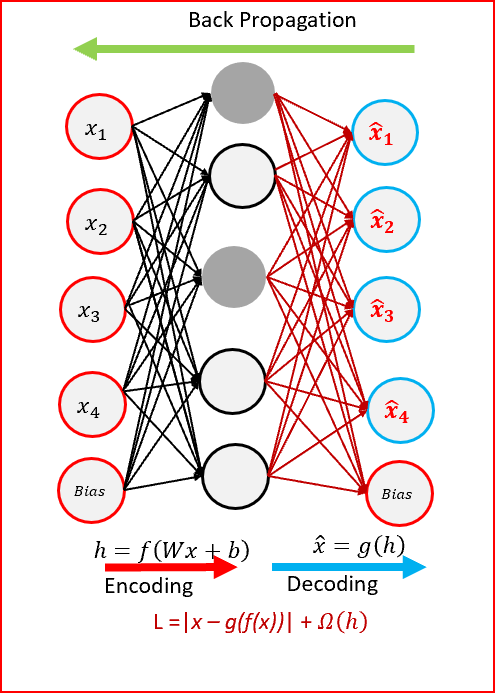Sparse Autoencoders use only reduced number of hidden nodes at a time
• Sparse autoencoders have hidden nodes greater than input nodes. They can still discover important features from the data.
• Sparsity constraint is introduced on the hidden layer. This is to prevent output layer copy input data.
• Sparse autoencoders have a sparsity penalty, Ω(h), a value close to zero but not zero. Sparsity penalty is applied on the hidden layer in addition to the reconstruction error. This prevents overfitting.
• Sparse autoencoders take the highest activation values in the hidden layer and zero out the rest of the hidden nodes. This prevents autoencoders to use all of the hidden nodes at a time and forcing only a reduced number of hidden nodes to be used.
• As we activate and inactivate hidden nodes for each row in the dataset. Each hidden node extracts a feature from the data

#### Denoising Autoencoders(DAE)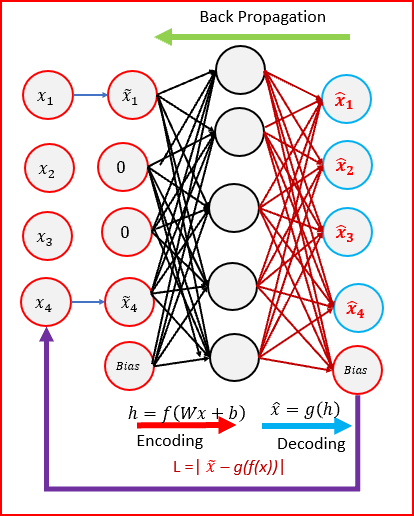Denoising Autoencoders — input is corrupted
• Denoising refers to intentionally adding noise to the raw input before providing it to the network. Denoising can be achieved using stochastic mapping.
• Denoising autoencoders create a corrupted copy of the input by introducing some noise. This helps to avoid the autoencoders to copy the input to the output without learning features about the data.
• Corruption of the input can be done randomly by making some of the input as zero. Remaining nodes copy the input to the noised input.
• Denoising autoencoders must remove the corruption to generate an output that is similar to the input. Output is compared with input and not with noised input. To minimize the loss function we continue until convergence
• Denoising autoencoders minimizes the loss function between the output node and the corrupted input.
• Denoising helps the autoencoders to learn the latent representation present in the data. Denoising autoencoders ensures a good representation is one that can be derived robustly from a corrupted input and that will be useful for recovering the corresponding clean input.
• Denoising is a stochastic autoencoder as we use a stochastic corruption process to set some of the inputs to zero

#### Contractive Autoencoders(CAE)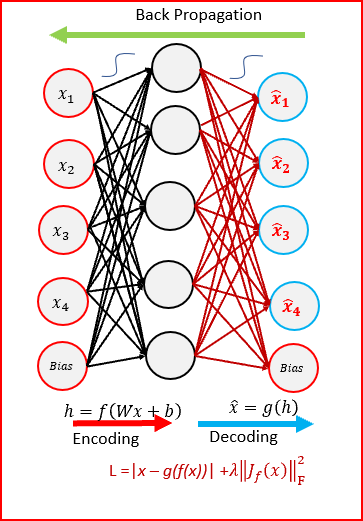Contractive Autoencoders
• Contractive autoencoder(CAE) objective is to have a robust learned representation which is less sensitive to small variation in the data.
• Robustness of the representation for the data is done by applying a penalty term to the loss function. The penalty term is Frobenius norm of the Jacobian matrix. Frobenius norm of the Jacobian matrix for the hidden layer is calculated with respect to input. Frobenius norm of the Jacobian matrix is the sum of square of all elements.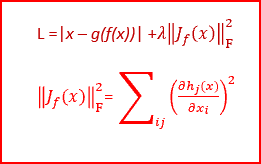Loss function with penalty term — Frobenius norm of the Jacobian matrix
• Contractive autoencoder is another regularization technique like sparse autoencoders and denoising autoencoders.
• CAE surpasses results obtained by regularizing autoencoder using weight decay or by denoising. CAE is a better choice than denoising autoencoder to learn useful feature extraction.
• Penalty term generates mapping which are strongly contracting the data and hence the name contractive autoencoder.

#### Stacked Denoising Autoencoders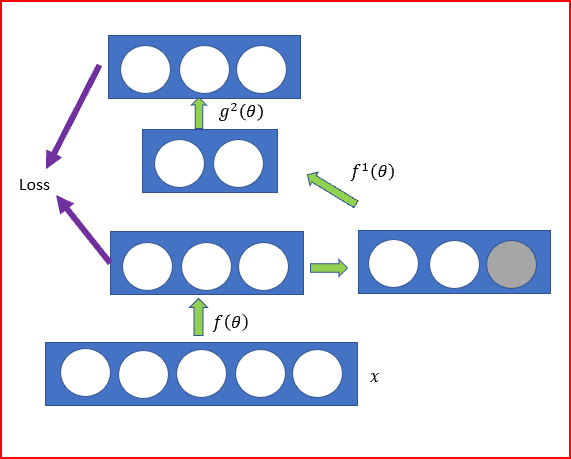Stacked Denoising Autoencoder
• Stacked Autoencoders is a neural network with multiple layers of sparse autoencoders
• When we add more hidden layers than just one hidden layer to an autoencoder, it helps to reduce a high dimensional data to a smaller code representing important features
• Each hidden layer is a more compact representation than the last hidden layer
• We can also denoise the input and then pass the data through the stacked autoencoders called as stacked denoising autoencoders
• In Stacked Denoising Autoencoders, input corruption is used only for initial denoising. This helps learn important features present in the data. Once the mapping function f(θ) has been learnt. For further layers we use uncorrupted input from the previous layers.
• After training a stack of encoders as explained above, we can use the output of the stacked denoising autoencoders as an input to a stand alone supervised machine learning like support vector machines or multi class logistics regression.

#### Deep Autoencoders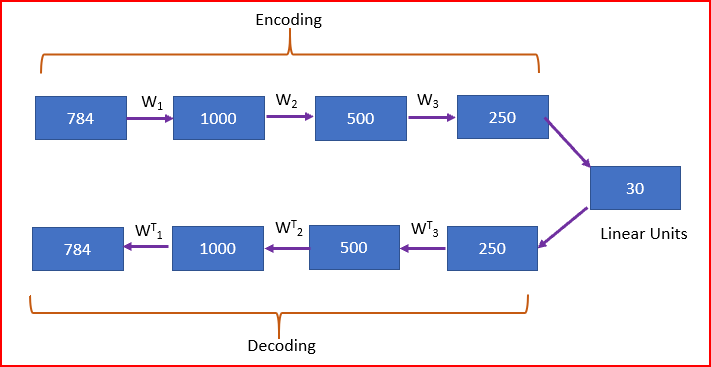Deep Autoencoders (Source: G. E. Hinton* and R. R. Salakhutdinov, Science , 2006)
• Deep Autoencoders consist of two identical deep belief networks. One network for encoding and another for decoding
• Typically deep autoencoders have 4 to 5 layers for encoding and the next 4 to 5 layers for decoding. We use unsupervised layer by layer pre-training
• Restricted Boltzmann Machine(RBM) is the basic building block of the deep belief network. We will do RBM is a different post.
• In the above figure, we take an image with 784 pixel. Train using a stack of 4 RBMs, unroll them and then finetune with back propagation
• Final encoding layer is compact and fast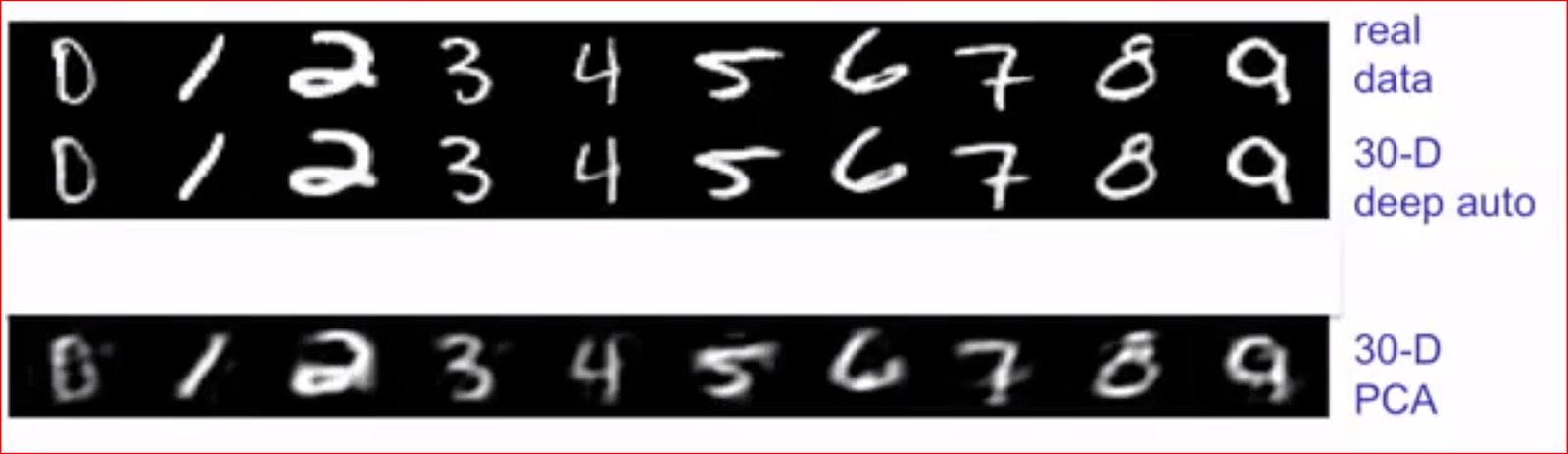Reconstructions by the 30-dimensional deep autoencoder; reconstructions by 30- dimensional standard PCA. (Source: G. E. Hinton* and R. R. Salakhutdinov, Science , 2006)

References:

Deep learning by Ian Goodfellow and Yoshua Bengio and Aaron Courville

http://www.icml-2011.org/papers/455_icmlpaper.pdf

http://www.jmlr.org/papers/volume11/vincent10a/vincent10a.pdf

### Share it and Clap if you liked the article!

Also published on mc.ai on December 2, 2018.